# Measure space

(diff) ← Older revision | Latest revision (diff) | Newer revision → (diff)A measurable space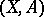with a measuregiven on(i.e. a countably-additive functionwith values infor which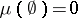; the latter property follows from additivity if the measure is finite, i.e. does not take the value, or even if there is some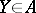with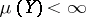). The notation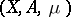is often shortened toand one says thatis a measure on; sometimes the notation is shortened to. The basic case is whenis a-algebra (cf. Algebra of sets) andcan be represented as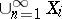with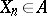and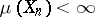. In this case the measure is called (totally)-finite (while if, then it is called (totally) finite). Such is, e.g., the Lebesgue measure on(cf. Lebesgue space). However, sometimes non--finite measures are encountered, such as, e.g., the-dimensional Hausdorff measure onfor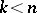. One may also encounter modifications in whichtakes values in, or complex or vector values, as well as cases whenis only finitely additive.